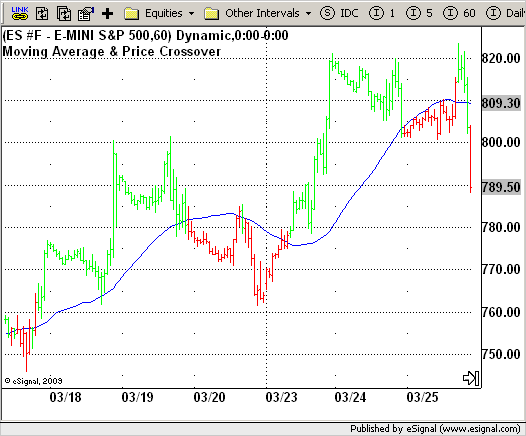# Moving Average & Price Crossover

ICE Data Services -

strMA_Pcrsv.efs
EFSLibrary - Discussion Board

File Name: strMA_Pcrsv.efs

Description:
Moving Average & Price Crossover

Formula Parameters:

• Length : 40

Notes:

This is the almost simpliest strategy a trader can imagine. It is based on moving average concept. A Moving Average is an indicator that shows the average value of a security's price over a period of
time. When calculating a moving average, a mathematical analysis of the security's average value over a predetermined time period is made. As the security's price changes, its average price moves up or down.

strMA_Pcrsv.efsEFS Code:

```/*********************************
Provided By:
eSignal (Copyright c eSignal), a division of Interactive Data
Formula Script (EFS) is for educational purposes only and may be
modified and saved under a new file name.  eSignal is not responsible
for the functionality once modified.  eSignal reserves the right
to modify and overwrite this EFS file with each new release.

Description:
Moving Average & Price Crossover

Version:            1.0  03/24/2009

Formula Parameters:                     Default:
Length                              40

Notes:
This is the almost simpliest strategy a trader can imagine. It is
based on moving average concept. A Moving Average is an indicator
that shows the average value of a security's price over a period of
time. When calculating a moving average, a mathematical analysis of
the security's average value over a predetermined time period is made.
As the security's price changes, its average price moves up or down.
**********************************/

var fpArray = new Array();
var bInit = false;

function preMain() {
setPriceStudy(true);
setStudyTitle("Moving Average & Price Crossover");
setCursorLabelName("SMA", 0);
setColorPriceBars(true);
setDefaultPriceBarColor(Color.black);

var x=0;
fpArray[x] = new FunctionParameter("Length", FunctionParameter.NUMBER);
with(fpArray[x++]){
setLowerLimit(1);
setDefault(40);
}
}

var xSMA = null;

function main(Length) {
var nBarState = getBarState();
var Close = close(-1);
var nSMA = 0;
if (nBarState == BARSTATE_ALLBARS) {
if (Length == null) nLength = 40;
}
if (bInit == false) {
xSMA = sma(Length);
bInit = true;
}

nSMA = xSMA.getValue(-1);
if (nSMA == null) return;

if (getCurrentBarIndex() == 0) return;

if(Close >= nSMA && !Strategy.isLong())
Strategy.doLong("Crossing Up", Strategy.MARKET, Strategy.THISBAR);
if(Close < nSMA && !Strategy.isShort())
Strategy.doShort("Crossing Down", Strategy.MARKET, Strategy.THISBAR);
if(Strategy.isLong())
setPriceBarColor(Color.lime);
else if(Strategy.isShort())
setPriceBarColor(Color.red);
return nSMA;
}```